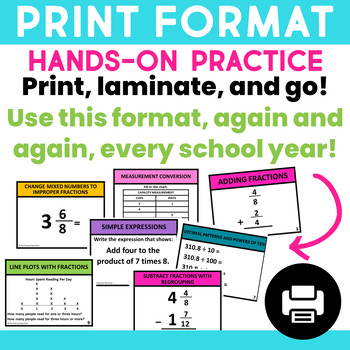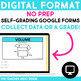Subject
Resource Type
File Type
Zip (42 MB|450 +)
Standards
\$48.00
Bundle
List Price:
\$94.25
You Save:
\$46.25
Products in this Bundle (30)

showing 1-5 of 30 products

• Bundle Description
• Standards

This 5th Grade Math Task Cards Mega Bundle contains 30 sets, for a total of 960 task cards which cover ALL MATH STANDARDS for 5th grade for the entire year! The task cards may be used for math centers, as whole class games, for one on one, as exit slips, and more! This resource is Common Core aligned but will work well in any classroom (there are no Common Core notations on the task cards).

Here’s what it includes:

Record Sheet for each set

Self-Checking Answer Sheets for each set

Teacher Notes

Labels for Photo Boxes (2 versions)

This bundle is deeply discounted so you can SAVE MORE and GET MORE!!!

Why you'll love this resource:

• Each task card set is aligned to a math standard to give your students effective, focused math practice.
• The task cards are easy to prep and to organize! The sets come with a variety of different colored or patterned borders.
• Students love the math task card format which seems less overwhelming but provides repeated reinforcement.
• Having a set of task cards for every math concept allows you to easily spiral math concepts throughout the year.
• The cards can be used in so many ways, from math centers, to whole class game times, one on one remediation, SCOOT games and scavenger hunts, exit slips, transition times, test prep, and more!!!

Here are the topics included in this bundle if you'd like to see one in more detail:

Order of Operations

Simple Expressions

Number Patterns

Decimals and Powers of Ten

Rounding Decimals

Multiply Whole Numbers

Multiply Decimals

Divide Whole Numbers

Divide Decimals by 1 Digit Divisors

Divide Decimals by 2 Digit Divisors

Problem Solving Decimals

Change Mixed Numbers to Improper Fractions

Change Improper Fractions to Mixed Numbers

Subtract Fractions

Subtract Fractions with Regrouping

Addition and Subtraction Fractions: Problem Solving

Multiply Fractions

Finding Area With Fractions

Dividing Fractions

Problem Solving Fractions (Multiplication and Division)

Measurement Conversion

Line Plot With Fractions

Volume

Problem Solving Volume

Coordinate Plane

Two Dimensional Figures

Mixed Review

The task card sets also come in smaller bundles, grouped by concept standards:

Operations and Algebraic Thinking Bundle (3 task card sets)

Number and Operations in Base Ten Bundle (10 sets of task cards)

Number and Operations - Fractions Bundle (10 sets of task cards)

Measurement and Data Bundle (4 task card sets)

Geometry Bundle (2 sets of task cards)

Looking for other year long resources for 5th grade?

20 Fiction and Nonfiction Games

30 Grammar Games

Comprehensive Morning Work/Homework for 5th Grade

Character Education Kit

Finally, if you like my work, I would love for you to follow me by clicking on the green star above.

Thanks so much,

Jenn

Purchasing this product grants permission for use by one teacher in his or her own classroom or for one homeschool parent teaching his/her child. If you would like to share with others, please purchase additional licenses.

Classify two-dimensional figures in a hierarchy based on properties.
Understand that attributes belonging to a category of two-dimensional figures also belong to all subcategories of that category. For example, all rectangles have four right angles and squares are rectangles, so all squares have four right angles.
Represent real world and mathematical problems by graphing points in the first quadrant of the coordinate plane, and interpret coordinate values of points in the context of the situation.
Use a pair of perpendicular number lines, called axes, to define a coordinate system, with the intersection of the lines (the origin) arranged to coincide with the 0 on each line and a given point in the plane located by using an ordered pair of numbers, called its coordinates. Understand that the first number indicates how far to travel from the origin in the direction of one axis, and the second number indicates how far to travel in the direction of the second axis, with the convention that the names of the two axes and the coordinates correspond (e.g., 𝘹-axis and 𝘹-coordinate, 𝘺-axis and 𝘺-coordinate).
Solve real world problems involving division of unit fractions by non-zero whole numbers and division of whole numbers by unit fractions, e.g., by using visual fraction models and equations to represent the problem. For example, how much chocolate will each person get if 3 people share 1/2 lb of chocolate equally? How many 1/3-cup servings are in 2 cups of raisins?
Total Pages
450 +